Home » Commonly used Machine Learning Algorithms (with Python and R Codes)

# Commonly used Machine Learning Algorithms (with Python and R Codes)

Note: This article was originally published on August 10, 2015 and updated on Sept 9th, 2017

## Overview

• Major focus on commonly used machine learning algorithms
• Algorithms covered- Linear regression, logistic regression, Naive Bayes, kNN, Random forest, etc.
• Learn both theory and implementation of these algorithms in R and python

## Introduction

Google’s self-driving cars and robots get a lot of press, but the company’s real future is in machine learning, the technology that enables computers to get smarter and more personal.

We are probably living in the most defining period of human history. The period when computing moved from large mainframes to PCs to the cloud. But what makes it defining is not what has happened, but what is coming our way in years to come.

What makes this period exciting and enthralling for someone like me is the democratization of the various tools and techniques, which followed the boost in computing. Welcome to the world of data science!

Today, as a data scientist, I can build data-crunching machines with complex algorithms for a few dollars per hour. But reaching here wasn’t easy! I had my dark days and nights.

Are you a beginner looking for a place to start your data science journey? Presenting two comprehensive courses, full of knowledge and data science learning, curated just for you to learn data science (using Python) from scratch:

## Who can benefit the most from this guide?

#### What I am giving out today is probably the most valuable guide, I have ever created.

The idea behind creating this guide is to simplify the journey of aspiring data scientists and machine learning enthusiasts across the world. Through this guide, I will enable you to work on machine learning problems and gain from experience. I am providing a high-level understanding of various machine learning algorithms along with R & Python codes to run them. These should be sufficient to get your hands dirty. You can also check out our Machine Learning Course.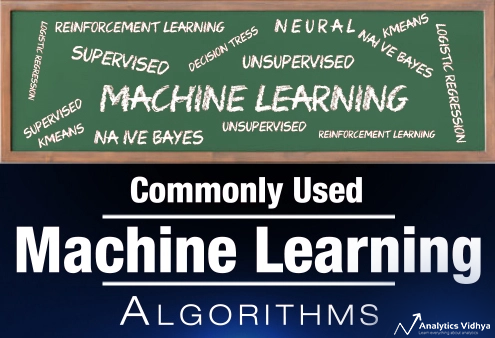Essentials of machine learning algorithms with implementation in R and Python

I have deliberately skipped the statistics behind these techniques, as you don’t need to understand them at the start. So, if you are looking for a statistical understanding of these algorithms, you should look elsewhere. But, if you are looking to equip yourself to start building a machine learning project, you are in for a treat.

## Broadly, there are 3 types of Machine Learning Algorithms

### 1. Supervised Learning

How it works: This algorithm consist of a target / outcome variable (or dependent variable) which is to be predicted from a given set of predictors (independent variables). Using these set of variables, we generate a function that map inputs to desired outputs. The training process continues until the model achieves a desired level of accuracy on the training data. Examples of Supervised Learning: Regression, Decision Tree, Random Forest, KNN, Logistic Regression etc.

### 2. Unsupervised Learning

How it works: In this algorithm, we do not have any target or outcome variable to predict / estimate. It is used for clustering population in different groups, which is widely used for segmenting customers in different groups for specific intervention. Examples of Unsupervised Learning: Apriori algorithm, K-means.

### 3. Reinforcement Learning:

How it works: Using this algorithm, the machine is trained to make specific decisions. It works this way: the machine is exposed to an environment where it trains itself continually using trial and error. This machine learns from past experience and tries to capture the best possible knowledge to make accurate business decisions. Example of Reinforcement Learning: Markov Decision Process

## List of Common Machine Learning Algorithms

Here is the list of commonly used machine learning algorithms. These algorithms can be applied to almost any data problem:

1. Linear Regression
2. Logistic Regression
3. Decision Tree
4. SVM
5. Naive Bayes
6. kNN
7. K-Means
8. Random Forest
9. Dimensionality Reduction Algorithms
1. GBM
2. XGBoost
3. LightGBM
4. CatBoost

## 1. Linear Regression

It is used to estimate real values (cost of houses, number of calls, total sales etc.) based on continuous variable(s). Here, we establish relationship between independent and dependent variables by fitting a best line. This best fit line is known as regression line and represented by a linear equation Y= a *X + b.

The best way to understand linear regression is to relive this experience of childhood. Let us say, you ask a child in fifth grade to arrange people in his class by increasing order of weight, without asking them their weights! What do you think the child will do? He / she would likely look (visually analyze) at the height and build of people and arrange them using a combination of these visible parameters. This is linear regression in real life! The child has actually figured out that height and build would be correlated to the weight by a relationship, which looks like the equation above.

In this equation:

• Y – Dependent Variable
• a – Slope
• X – Independent variable
• b – Intercept

These coefficients a and b are derived based on minimizing the sum of squared difference of distance between data points and regression line.

Look at the below example. Here we have identified the best fit line having linear equation y=0.2811x+13.9. Now using this equation, we can find the weight, knowing the height of a person.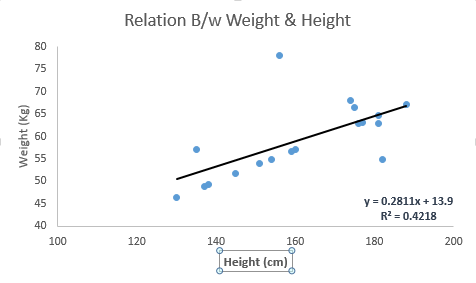Linear Regression is mainly of two types: Simple Linear Regression and Multiple Linear Regression. Simple Linear Regression is characterized by one independent variable. And, Multiple Linear Regression(as the name suggests) is characterized by multiple (more than 1) independent variables. While finding the best fit line, you can fit a polynomial or curvilinear regression. And these are known as polynomial or curvilinear regression.

Here’s a coding window to try out your hand and build your own linear regression model in Python:

R Code

```#Load Train and Test datasets
#Identify feature and response variable(s) and values must be numeric and numpy arrays
x_train <- input_variables_values_training_datasets
y_train <- target_variables_values_training_datasets
x_test <- input_variables_values_test_datasets
x <- cbind(x_train,y_train)
# Train the model using the training sets and check score
linear <- lm(y_train ~ ., data = x)
summary(linear)
#Predict Output
predicted= predict(linear,x_test)```

## 2. Logistic Regression

Don’t get confused by its name! It is a classification not a regression algorithm. It is used to estimate discrete values ( Binary values like 0/1, yes/no, true/false ) based on given set of independent variable(s). In simple words, it predicts the probability of occurrence of an event by fitting data to a logit function. Hence, it is also known as logit regression. Since, it predicts the probability, its output values lies between 0 and 1 (as expected).

Again, let us try and understand this through a simple example.

Let’s say your friend gives you a puzzle to solve. There are only 2 outcome scenarios – either you solve it or you don’t. Now imagine, that you are being given wide range of puzzles / quizzes in an attempt to understand which subjects you are good at. The outcome to this study would be something like this – if you are given a trignometry based tenth grade problem, you are 70% likely to solve it. On the other hand, if it is grade fifth history question, the probability of getting an answer is only 30%. This is what Logistic Regression provides you.

Coming to the math, the log odds of the outcome is modeled as a linear combination of the predictor variables.

```odds= p/ (1-p) = probability of event occurrence / probability of not event occurrence
ln(odds) = ln(p/(1-p))
logit(p) = ln(p/(1-p)) = b0+b1X1+b2X2+b3X3....+bkXk```

Above, p is the probability of presence of the characteristic of interest. It chooses parameters that maximize the likelihood of observing the sample values rather than that minimize the sum of squared errors (like in ordinary regression).

Now, you may ask, why take a log? For the sake of simplicity, let’s just say that this is one of the best mathematical way to replicate a step function. I can go in more details, but that will beat the purpose of this article.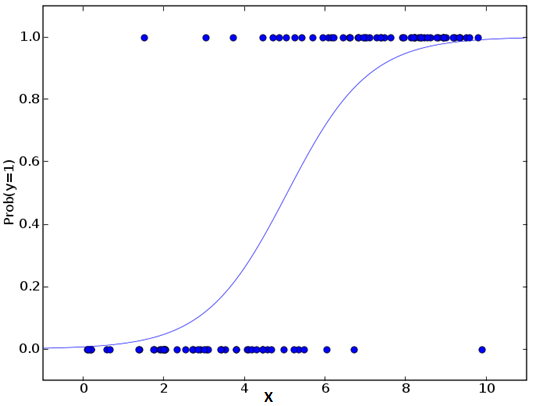Build your own logistic regression model in Python here and check the accuracy:

R Code

```x <- cbind(x_train,y_train)
# Train the model using the training sets and check score
logistic <- glm(y_train ~ ., data = x,family='binomial')
summary(logistic)
#Predict Output
predicted= predict(logistic,x_test)```

### Furthermore..

There are many different steps that could be tried in order to improve the model:

## 3. Decision Tree

This is one of my favorite algorithm and I use it quite frequently. It is a type of supervised learning algorithm that is mostly used for classification problems. Surprisingly, it works for both categorical and continuous dependent variables. In this algorithm, we split the population into two or more homogeneous sets. This is done based on most significant attributes/ independent variables to make as distinct groups as possible. For more details, you can read: Decision Tree Simplified.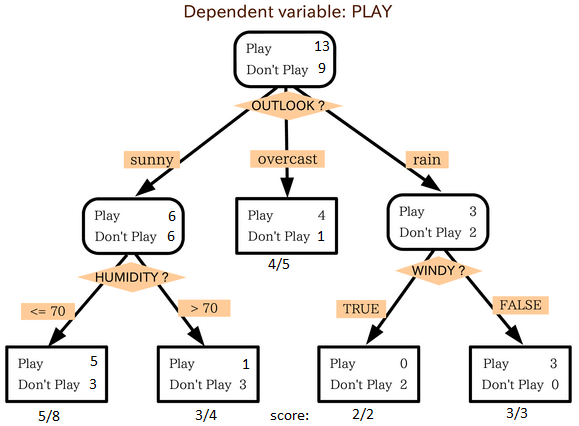source: statsexchange

In the image above, you can see that population is classified into four different groups based on multiple attributes to identify ‘if they will play or not’. To split the population into different heterogeneous groups, it uses various techniques like Gini, Information Gain, Chi-square, entropy.

The best way to understand how decision tree works, is to play Jezzball – a classic game from Microsoft (image below). Essentially, you have a room with moving walls and you need to create walls such that maximum area gets cleared off with out the balls.So, every time you split the room with a wall, you are trying to create 2 different populations with in the same room. Decision trees work in very similar fashion by dividing a population in as different groups as possible.

Let’s get our hands dirty and code our own decision tree in Python!

R Code

```library(rpart)
x <- cbind(x_train,y_train)
# grow tree
fit <- rpart(y_train ~ ., data = x,method="class")
summary(fit)
#Predict Output
predicted= predict(fit,x_test)```

## 4. SVM (Support Vector Machine)

It is a classification method. In this algorithm, we plot each data item as a point in n-dimensional space (where n is number of features you have) with the value of each feature being the value of a particular coordinate.

For example, if we only had two features like Height and Hair length of an individual, we’d first plot these two variables in two dimensional space where each point has two co-ordinates (these co-ordinates are known as Support Vectors)Now, we will find some line that splits the data between the two differently classified groups of data. This will be the line such that the distances from the closest point in each of the two groups will be farthest away.In the example shown above, the line which splits the data into two differently classified groups is the black line, since the two closest points are the farthest apart from the line. This line is our classifier. Then, depending on where the testing data lands on either side of the line, that’s what class we can classify the new data as.

Think of this algorithm as playing JezzBall in n-dimensional space. The tweaks in the game are:

• You can draw lines/planes at any angles (rather than just horizontal or vertical as in the classic game)
• The objective of the game is to segregate balls of different colors in different rooms.
• And the balls are not moving.

Try your hand and design an SVM model in Python through this coding window:

R Code

```library(e1071)
x <- cbind(x_train,y_train)
# Fitting model
fit <-svm(y_train ~ ., data = x)
summary(fit)
#Predict Output
predicted= predict(fit,x_test)```

## 5. Naive Bayes

It is a classification technique based on Bayes’ theorem with an assumption of independence between predictors. In simple terms, a Naive Bayes classifier assumes that the presence of a particular feature in a class is unrelated to the presence of any other feature. For example, a fruit may be considered to be an apple if it is red, round, and about 3 inches in diameter. Even if these features depend on each other or upon the existence of the other features, a naive Bayes classifier would consider all of these properties to independently contribute to the probability that this fruit is an apple.

Naive Bayesian model is easy to build and particularly useful for very large data sets. Along with simplicity, Naive Bayes is known to outperform even highly sophisticated classification methods.

Bayes theorem provides a way of calculating posterior probability P(c|x) from P(c), P(x) and P(x|c). Look at the equation below: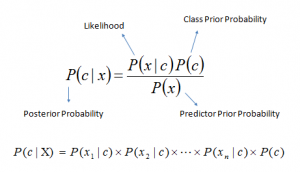Here,

• P(c|x) is the posterior probability of class (target) given predictor (attribute).
• P(c) is the prior probability of class.
• P(x|c) is the likelihood which is the probability of predictor given class.
• P(x) is the prior probability of predictor.

Example: Let’s understand it using an example. Below I have a training data set of weather and corresponding target variable ‘Play’. Now, we need to classify whether players will play or not based on weather condition. Let’s follow the below steps to perform it.

Step 1: Convert the data set to frequency table

Step 2: Create Likelihood table by finding the probabilities like Overcast probability = 0.29 and probability of playing is 0.64.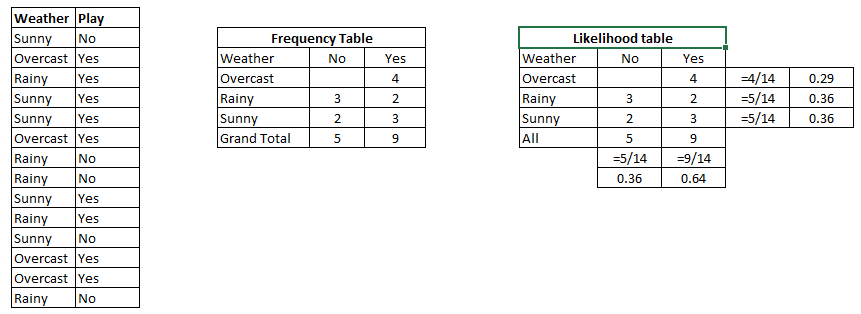Step 3: Now, use Naive Bayesian equation to calculate the posterior probability for each class. The class with the highest posterior probability is the outcome of prediction.

Problem: Players will pay if weather is sunny, is this statement is correct?

We can solve it using above discussed method, so P(Yes | Sunny) = P( Sunny | Yes) * P(Yes) / P (Sunny)

Here we have P (Sunny |Yes) = 3/9 = 0.33, P(Sunny) = 5/14 = 0.36, P( Yes)= 9/14 = 0.64

Now, P (Yes | Sunny) = 0.33 * 0.64 / 0.36 = 0.60, which has higher probability.

Naive Bayes uses a similar method to predict the probability of different class based on various attributes. This algorithm is mostly used in text classification and with problems having multiple classes.

Code a Naive Bayes classification model in Python:

R Code

```library(e1071)
x <- cbind(x_train,y_train)
# Fitting model
fit <-naiveBayes(y_train ~ ., data = x)
summary(fit)
#Predict Output
predicted= predict(fit,x_test)```

## 6. kNN (k- Nearest Neighbors)

It can be used for both classification and regression problems. However, it is more widely used in classification problems in the industry. K nearest neighbors is a simple algorithm that stores all available cases and classifies new cases by a majority vote of its k neighbors. The case being assigned to the class is most common amongst its K nearest neighbors measured by a distance function.

These distance functions can be Euclidean, Manhattan, Minkowski and Hamming distance. First three functions are used for continuous function and fourth one (Hamming) for categorical variables. If K = 1, then the case is simply assigned to the class of its nearest neighbor. At times, choosing K turns out to be a challenge while performing kNN modeling.KNN can easily be mapped to our real lives. If you want to learn about a person, of whom you have no information, you might like to find out about his close friends and the circles he moves in and gain access to his/her information!

Things to consider before selecting kNN:

• KNN is computationally expensive
• Variables should be normalized else higher range variables can bias it
• Works on pre-processing stage more before going for kNN like an outlier, noise removal

#### Python Code

R Code

```library(knn)
x <- cbind(x_train,y_train)
# Fitting model
fit <-knn(y_train ~ ., data = x,k=5)
summary(fit)
#Predict Output
predicted= predict(fit,x_test)```

## 7. K-Means

It is a type of unsupervised algorithm which solves the clustering problem. Its procedure follows a simple and easy way to classify a given data set through a certain number of clusters (assume k clusters). Data points inside a cluster are homogeneous and heterogeneous to peer groups.

Remember figuring out shapes from ink blots? k means is somewhat similar this activity. You look at the shape and spread to decipher how many different clusters / population are present!How K-means forms cluster:

1. K-means picks k number of points for each cluster known as centroids.
2. Each data point forms a cluster with the closest centroids i.e. k clusters.
3. Finds the centroid of each cluster based on existing cluster members. Here we have new centroids.
4. As we have new centroids, repeat step 2 and 3. Find the closest distance for each data point from new centroids and get associated with new k-clusters. Repeat this process until convergence occurs i.e. centroids does not change.

How to determine value of K:

In K-means, we have clusters and each cluster has its own centroid. Sum of square of difference between centroid and the data points within a cluster constitutes within sum of square value for that cluster. Also, when the sum of square values for all the clusters are added, it becomes total within sum of square value for the cluster solution.

We know that as the number of cluster increases, this value keeps on decreasing but if you plot the result you may see that the sum of squared distance decreases sharply up to some value of k, and then much more slowly after that. Here, we can find the optimum number of cluster.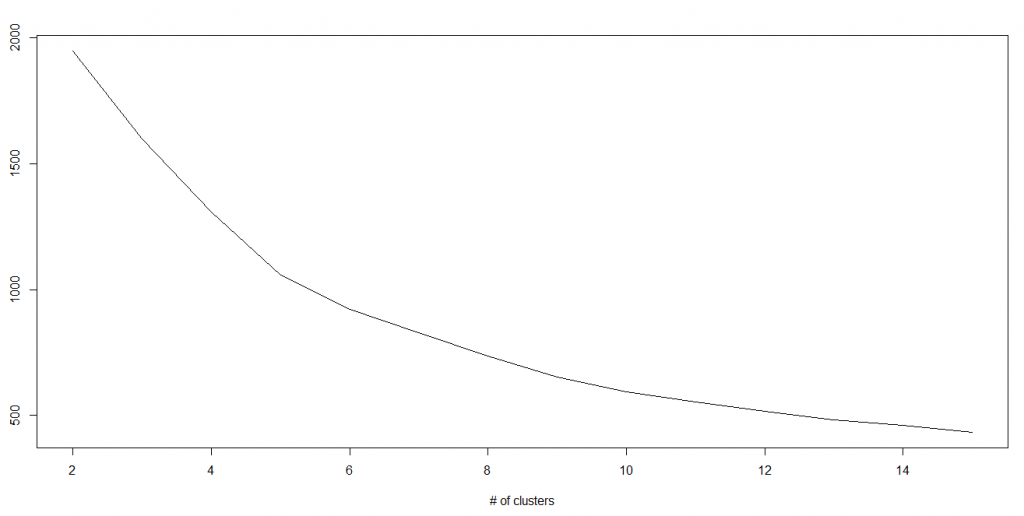#### Python Code

R Code

```library(cluster)
fit <- kmeans(X, 3) # 5 cluster solution```

## 8. Random Forest

Random Forest is a trademark term for an ensemble of decision trees. In Random Forest, we’ve collection of decision trees (so known as “Forest”). To classify a new object based on attributes, each tree gives a classification and we say the tree “votes” for that class. The forest chooses the classification having the most votes (over all the trees in the forest).

Each tree is planted & grown as follows:

1. If the number of cases in the training set is N, then sample of N cases is taken at random but with replacement. This sample will be the training set for growing the tree.
2. If there are M input variables, a number m<<M is specified such that at each node, m variables are selected at random out of the M and the best split on these m is used to split the node. The value of m is held constant during the forest growing.
3. Each tree is grown to the largest extent possible. There is no pruning.

For more details on this algorithm, comparing with decision tree and tuning model parameters, I would suggest you to read these articles:

Python Code:

R Code

```library(randomForest)
x <- cbind(x_train,y_train)
# Fitting model
fit <- randomForest(Species ~ ., x,ntree=500)
summary(fit)
#Predict Output
predicted= predict(fit,x_test)```

## 9. Dimensionality Reduction Algorithms

In the last 4-5 years, there has been an exponential increase in data capturing at every possible stages. Corporates/ Government Agencies/ Research organisations are not only coming with new sources but also they are capturing data in great detail.

For example: E-commerce companies are capturing more details about customer like their demographics, web crawling history, what they like or dislike, purchase history, feedback and many others to give them personalized attention more than your nearest grocery shopkeeper.

As a data scientist, the data we are offered also consist of many features, this sounds good for building good robust model but there is a challenge. How’d you identify highly significant variable(s) out 1000 or 2000? In such cases, dimensionality reduction algorithm helps us along with various other algorithms like Decision Tree, Random Forest, PCA, Factor Analysis, Identify based on correlation matrix, missing value ratio and others.

#### R Code

```library(stats)
pca <- princomp(train, cor = TRUE)
train_reduced  <- predict(pca,train)
test_reduced  <- predict(pca,test)```

### 10.1. GBM

GBM is a boosting algorithm used when we deal with plenty of data to make a prediction with high prediction power. Boosting is actually an ensemble of learning algorithms which combines the prediction of several base estimators in order to improve robustness over a single estimator. It combines multiple weak or average predictors to a build strong predictor. These boosting algorithms always work well in data science competitions like Kaggle, AV Hackathon, CrowdAnalytix.

#### R Code

```library(caret)
x <- cbind(x_train,y_train)
# Fitting model
fitControl <- trainControl( method = "repeatedcv", number = 4, repeats = 4)
fit <- train(y ~ ., data = x, method = "gbm", trControl = fitControl,verbose = FALSE)
predicted= predict(fit,x_test,type= "prob")[,2] ```

GradientBoostingClassifier and Random Forest are two different boosting tree classifier and often people ask about the difference between these two algorithms.

### 10.2. XGBoost

Another classic gradient boosting algorithm that’s known to be the decisive choice between winning and losing in some Kaggle competitions.

The XGBoost has an immensely high predictive power which makes it the best choice for accuracy in events as it possesses both linear model and the tree learning algorithm, making the algorithm almost 10x faster than existing gradient booster techniques.

The support includes various objective functions, including regression, classification and ranking.

One of the most interesting things about the XGBoost is that it is also called a regularized boosting technique. This helps to reduce overfit modelling and has a massive support for a range of languages such as Scala, Java, R, Python, Julia and C++.

Supports distributed and widespread training on many machines that encompass GCE, AWS, Azure and Yarn clusters. XGBoost can also be integrated with Spark, Flink and other cloud dataflow systems with a built in cross validation at each iteration of the boosting process.

Python Code:

R Code:

```require(caret)

x <- cbind(x_train,y_train)

# Fitting model

TrainControl <- trainControl( method = "repeatedcv", number = 10, repeats = 4)

model<- train(y ~ ., data = x, method = "xgbLinear", trControl = TrainControl,verbose = FALSE)

OR

model<- train(y ~ ., data = x, method = "xgbTree", trControl = TrainControl,verbose = FALSE)

predicted <- predict(model, x_test)```

### 10.3. LightGBM

LightGBM is a gradient boosting framework that uses tree based learning algorithms. It is designed to be distributed and efficient with the following advantages:

• Faster training speed and higher efficiency
• Lower memory usage
• Better accuracy
• Parallel and GPU learning supported
• Capable of handling large-scale data

The framework is a fast and high-performance gradient boosting one based on decision tree algorithms, used for ranking, classification and many other machine learning tasks. It was developed under the Distributed Machine Learning Toolkit Project of Microsoft.

Since the LightGBM is based on decision tree algorithms, it splits the tree leaf wise with the best fit whereas other boosting algorithms split the tree depth wise or level wise rather than leaf-wise. So when growing on the same leaf in Light GBM, the leaf-wise algorithm can reduce more loss than the level-wise algorithm and hence results in much better accuracy which can rarely be achieved by any of the existing boosting algorithms.

Also, it is surprisingly very fast, hence the word ‘Light’.

Refer to the article to know more about LightGBM: https://www.analyticsvidhya.com/blog/2017/06/which-algorithm-takes-the-crown-light-gbm-vs-xgboost/

Python Code:

```data = np.random.rand(500, 10) # 500 entities, each contains 10 features
label = np.random.randint(2, size=500) # binary target

train_data = lgb.Dataset(data, label=label)
test_data = train_data.create_valid('test.svm')

param = {'num_leaves':31, 'num_trees':100, 'objective':'binary'}
param['metric'] = 'auc'

num_round = 10
bst = lgb.train(param, train_data, num_round, valid_sets=[test_data])

bst.save_model('model.txt')

# 7 entities, each contains 10 features
data = np.random.rand(7, 10)
ypred = bst.predict(data)

```

R Code:

```library(RLightGBM)
data(example.binary)
#Parameters

num_iterations <- 100
config <- list(objective = "binary",  metric="binary_logloss,auc", learning_rate = 0.1, num_leaves = 63, tree_learner = "serial", feature_fraction = 0.8, bagging_freq = 5, bagging_fraction = 0.8, min_data_in_leaf = 50, min_sum_hessian_in_leaf = 5.0)

#Create data handle and booster
handle.data <- lgbm.data.create(x)

lgbm.data.setField(handle.data, "label", y)

handle.booster <- lgbm.booster.create(handle.data, lapply(config, as.character))

#Train for num_iterations iterations and eval every 5 steps

lgbm.booster.train(handle.booster, num_iterations, 5)

#Predict
pred <- lgbm.booster.predict(handle.booster, x.test)

#Test accuracy
sum(y.test == (y.pred > 0.5)) / length(y.test)

lgbm.booster.save(handle.booster, filename = "/tmp/model.txt")```

If you’re familiar with the Caret package in R, this is another way of implementing the LightGBM.

```require(caret)
require(RLightGBM)
data(iris)

model <-caretModel.LGBM()

fit <- train(Species ~ ., data = iris, method=model, verbosity = 0)
print(fit)
y.pred <- predict(fit, iris[,1:4])

library(Matrix)
model.sparse <- caretModel.LGBM.sparse()

#Generate a sparse matrix
mat <- Matrix(as.matrix(iris[,1:4]), sparse = T)
fit <- train(data.frame(idx = 1:nrow(iris)), iris\$Species, method = model.sparse, matrix = mat, verbosity = 0)
print(fit)

```

### 10.4. Catboost

CatBoost is a recently open-sourced machine learning algorithm from Yandex. It can easily integrate with deep learning frameworks like Google’s TensorFlow and Apple’s Core ML.

The best part about CatBoost is that it does not require extensive data training like other ML models, and can work on a variety of data formats; not undermining how robust it can be.

Make sure you handle missing data well before you proceed with the implementation.

Catboost can automatically deal with categorical variables without showing the type conversion error, which helps you to focus on tuning your model better rather than sorting out trivial errors.

Python Code:

```import pandas as pd
import numpy as np

from catboost import CatBoostRegressor

#Imputing missing values for both train and test
train.fillna(-999, inplace=True)
test.fillna(-999,inplace=True)

#Creating a training set for modeling and validation set to check model performance
X = train.drop(['Item_Outlet_Sales'], axis=1)
y = train.Item_Outlet_Sales

from sklearn.model_selection import train_test_split

X_train, X_validation, y_train, y_validation = train_test_split(X, y, train_size=0.7, random_state=1234)
categorical_features_indices = np.where(X.dtypes != np.float)

#importing library and building model
from catboost import CatBoostRegressormodel=CatBoostRegressor(iterations=50, depth=3, learning_rate=0.1, loss_function='RMSE')

model.fit(X_train, y_train,cat_features=categorical_features_indices,eval_set=(X_validation, y_validation),plot=True)

submission = pd.DataFrame()

submission['Item_Identifier'] = test['Item_Identifier']
submission['Outlet_Identifier'] = test['Outlet_Identifier']
submission['Item_Outlet_Sales'] = model.predict(test)```

R Code:

```set.seed(1)

require(titanic)

require(caret)

require(catboost)

tt <- titanic::titanic_train[complete.cases(titanic::titanic_train),]

data <- as.data.frame(as.matrix(tt), stringsAsFactors = TRUE)

drop_columns = c("PassengerId", "Survived", "Name", "Ticket", "Cabin")

x <- data[,!(names(data) %in% drop_columns)]y <- data[,c("Survived")]

fit_control <- trainControl(method = "cv", number = 4,classProbs = TRUE)

grid <- expand.grid(depth = c(4, 6, 8),learning_rate = 0.1,iterations = 100, l2_leaf_reg = 1e-3,            rsm = 0.95, border_count = 64)

report <- train(x, as.factor(make.names(y)),method = catboost.caret,verbose = TRUE, preProc = NULL,tuneGrid = grid, trControl = fit_control)

print(report)

importance <- varImp(report, scale = FALSE)

print(importance)```

## Projects

Now, its time to take the plunge and actually play with some other real datasets. So are you ready to take on the challenge? Accelerate your data science journey with the following Practice Problems:Practice Problem: Food Demand Forecasting Challenge Predict the demand of meals for a meal delivery companyPractice Problem: HR Analytics Challenge Identify the employees most likely to get promotedPractice Problem: Predict Number of Upvotes Predict number of upvotes on a query asked at an online question & answer platform

## End Notes

By now, I am sure, you would have an idea of commonly used machine learning algorithms. My sole intention behind writing this article and providing the codes in R and Python is to get you started right away. If you are keen to master machine learning, start right away. Take up problems, develop a physical understanding of the process, apply these codes and see the fun!

### If you like what you just read & want to continue your analytics learning, subscribe to our emails, follow us on twitter or like our facebook page.

You can also read this article on our Mobile APP•Kuber says:

Awesowe compilation!! Thank you.

•Karthikeyan says:

•hemanth says:

Straight, Informative and effective!!
Thank you

•venugopal says:

Good Summary airticle

•Dr Venugopala Rao says:

Super Compilation…

•Kishor Basyal says:

•Brian Thomas says:

Very nicely done! Thanks for this.

•Tesfaye says:

Thank you! Well presented article.

•Tesfaye says:

Thank you! Well presented.

•Huzefa says:

Hello,

Superb information in just one blog. Can anyone help me to run the codes in R what should be replaced with “~” symbol in codes? Help is appreciated

•Huzefa says:

Hello,

Superb information in just one blog. Can anyone help me to run the codes in R what should be replaced with “~” symbol in codes? Help is appreciated .

•AshuthoshGowda says:

“~” is used to select the variables that you’ll be using for a particular model.
“label~.,” – Uses all your Attributes
“label~Att1 + Att2,” – Uses only Att1 and Att2 to create the model

•Sudipta Basak says:

Enjoyed the simplicity. Thanks for the effort.

•Im_utm says:

Great Article… Helps a lot, as naive in Machine Learning.

•Sunil Ray says:

Hi All,

Thanks for the comment …

•Dalila says:

Very good summary.

Thank!
One simple point. The reason for taking the log(p/(1-p)) in Logistic Regression is to make the equation linear, I.e., easy to solve.

•Sunil Ray says:

Thanks Dalila… 🙂

•Borun Chowdhury says:

That’s not the reason for taking the log. The underlying assumption in logistic regression is that the probability is governed by a step function whose argument is linear in the attributes. First of all the assumption of linearity or otherwise introduces bias. However, logistic regression being a parametric model some bias is inevitable. The reason to choose a linear relationship is not because its easy to solve but because a higher order polynomial introduces higher bias and one would not like to do so without good reason.

Now coming to the choice of log, it is just a convention. Basically, once we have decided to go with a linear model, in the case of one attribute we model the probability by

p(x) = f( ax+b)

such that p(-infinity)=0 and p(infinity)=0. It so happens that this is satisfied by

p(x) = exp(ax+b)/ (1 + exp(ax+b))

which can be re-written as

log(p(x)/(1-p(x)) = a x+ b

While I am at it, it may be useful to talk about another point. One should ask is why we don’t use least square method. The reason is that a yes/no choice is a Bernoulli random variable and thus we estimate the probability according to maximum likelihood wrt Bernoulli process. For linear regression the assumption is that the residuals around the ‘true’ function are distributed according to a normal distribution and the maximum likelihood estimate for a normal distribution amounts to the least square method. So deep down linear regression and logistic regression both use maximum likelihood estimates. Its just that they are max likelihoods according to different distributions.

•Statis says:

Nice summary!
@Huzefa: you shouldn’t replace the “~” in the R code, it basically means “as a function of”. You can also keep the “.” right after, it stands for “all other variables in the dataset provided”. If you want to be explicit, you can write y ~ x1 + x2 + … where x1, x2 .. are the names of the columns of your data.frame or data.table.
Further note on formula specification: by default R adds an intercept, so that y ~ x is equivalent to y ~ 1 + x, you can remove it via y ~ 0 + x. Interactions are specified with either * (which also adds the two variables) or : (which only adds the interaction term). y ~ x1 * x2 is equivalent to y ~ x1 + x2 + x1 : x2.
Hope this helps!

•Chris says:

You did a Wonderful job! This is really helpful. Thanks!

•Glenn Nelson says:

I took the Stanford-Coursera ML class, but have not used it, and I found this to be an incredibly useful summary. I appreciate the real-world analogues, such as your mention of Jezzball. And showing the brief code snips is terrific.

•Shankar Pandala says:

This is very easy and helpful than any other courses I have completed.
simple. clear. To the point.

•markpratley says:

You Sir are a gentleman and a scholar!

•whystatistics says:

Hi Sunil,

This is really superb tutorial along with good examples and codes which is surely much helpful. Just, can you add Neural Network here in simple terms with example and code.

•Sayan Putatunda says:

Errata:- fit <- kmeans(X, 3) # 5 cluster solution
It`s a 3 cluster solution.

•Baha says:

Well done, Thank you!

•Benjamin says:

This is a great resource overall and surely the product of a lot of work.

Just a note as I go through this, your comment on Logistic Regression not actually being regression is in fact wrong. It maps outputs to a continuous variable bound between 0 and 1 that we regard as probability. it makes classification easy but that is still an extra step that requires the choice of a threshold which is not the main aim of Logistic Regression. As a matter of fact it falls under the umbrella of Generalized Libear Models as the glm R package hints it in your code example.

I thought this was interesting to note so as not to forget that logistic regression output is richer than 0 or 1.
Thanks for the great article overall.

•Ashish Yelkar says:

Very Nice.!!

•Bansari Shah says:

•ayushgg92 says:

I wanted to know if I can use rattle instead of writing the R code explicitly

•Debasis says:

Thank you. Very nice and useful article..

•Debashis says:

This is such a wonderful article.

•Anthony says:

Informative and easy to follow. I’ve recently started following several pages like this one and this is the best material ive seen yet.

•Akhil says:

One of the best content ever read regarding algorithms.

•Swathi says:

•Nícolas Robles says:

Cool stuff!
I just can’t get the necessary libraries…

•wizzerd says:

looks sgood article. Do I need any data to do the examples?

•Col. Dan Sulzinger says:

Good Article.

•Pansy says:

I have to thank you for this informative summary. Really useful!

•J says:

Somewhat irresponsible article since it does not mention any measure of performance and only gives cooking recipes without understanding what algorithm does what and the stats behind it. Cooking recipes like these are the ones that place people in Drew Conway’s danger zone (https://www.quora.com/In-the-data-science-venn-diagram-why-is-the-common-region-of-Hacking-Skills-and-Substantive-Expertise-considered-as-danger-zone), thus making programmers the worst data analysts (let alone scientists, that requires another mindset completely). I highly recommend anyone wishing to enter into this brave new world not to jump into statistical learning without proper statistical background. Otherwise you could end up like Google, Target, Telefonica, or Google (again) and become a poster boy for “The Big Flops of Big Data”.

•George says:

Do you have a better article?Please share…

•Robin White says:

Great article. It really summarize some of the most important topics on machine learning.
But as asked above I would like to present thedevmasters.com as a company with a really good course to learn more depth about machine learning with great professors and a sense of community that is always helping itself to continue learning even after the course ends.

•salman ahmed says:

•Borun Chowdhury says:

Very succinct description of some important algorithms. Thanks. I’d like to point out a mistake in the SVM section. You say “where each point has two co-ordinates (these co-ordinates are known as Support Vectors)”. This is not correct, the coordinates are just features. Its the points lying on the margin that are called the ‘support vectors’. These are the points that ‘support’ the margin i.e. define it (as opposed to a weighted average of all points for instance.)

•Isaac says:

Thank you for this wonderful article…it’s proven helpful.

•Payal gour says:

Thank you very much, A Very useful and excellent compilation.

•nd says:

Very good information interms of initial knowledge
Note one warning, many methods can be fitted into a particular problem, but result might not be what you wish.
Hence you must always compare models, understand residuals profile and how prediction really predicts.
In that sense, analysis of data is never ending.
In R, use summary, plot and check for assumptions validity .

•Dung Dinh says:

The amazing article. I’m new in data analysis. It’s very useful and easy to understand.

Thanks,

•sabarikannan says:

This is really good article, also if you would have explain about Anomaly dection algorithm that will really helpful for everyone to know , what and where to apply in machine learning….

•ankita srivastava says:

a very very helpful tutorial. Thanks a lot you guys.

•Haiyan says:

The amazing article

•Namala Santosh Kumar says:

Analytics Vidhya – I am loving it

•Mohammed Abdul Kaleem says:

Good article.
thank you for explaining with python.

•Jacques Gouimenou says:

very useful compilation. Thanks!

•Baseer says:

Very precise quick tutorial for those who want to gain insight of machine learning

•vishwas says:

great

•Ali Kazim says:

Very useful and informative. Thanks for sharing it.

•JS says:

Superb!

•Denis says:

great summary,
Thank you

•sanjiv says:

Great article. It would have become even better if you had some test data with each code snippet. Add metrics and hyper parameter tunning for each of these models

•Faizan says:

Thanks for the “jezzball” example. You made my day!

•Satya Swarup Dani says:

Nicely complied. Every explanation is crystal clear and very easy to digest. Thanks for sharing knowledge.

•Emerson Moizes says:

Perfect! It’s exactly what I was looking for!
Thanks for the explanation and thanks for sharing your knowledge with us!

•Valery says:

Very useful summary. Thank you.

•Malini Ramamurthy says:

Very informative article. For a person new to machine learning, this article gives a good starting point.

•Shubham says:

Good article.
thank you for explaining with python.

•Anand Rai says:

Good article.
thank you for explaining with python.

— Kareermatrix

•ramesh says:

Hi Friends, i’m new person to these machine learning algorithms. i have some questions.?
1) we have so many ML algorithms. but how can we choose the algorithms which one is suitable for my data set?
2) How does these algorithms works.?
3) why only these particular algorithms.? why not others.?

•Indra says:

Nice Article.. Thanks for your effort

•RAVI RATHORE says:

hello

I have to implement machine learning algorithms in python so could you help me in this.
any body provide me the proper code for any algorithm.

•Raghav says:

All programe has error named
Error in model.frame.default(formula = as.list(y_train) ~ ., data = x, :
invalid type (list) for variable ‘as.list(y_train)’

What is that ?

•Gaurav says:

I think that “y_train” is data frame and it cannot be converted directly to list with “as.list” command. Try this instead

y_train <- as.list(as.data.frame(t(y_train)))

See if this works for you.

•Suman says:

Very nice summary!

Can you tell how to get machine learning problems for practice?

•NSS says:

Analytics Vidhya has some practice datasets. Check Analytics Vidhya hackathon. Also UCI machine learning repository is a phenomenal place. Google it and enjoy.

•YB says:

Do you have R codes based on caret?

•NSS says:

Yes.